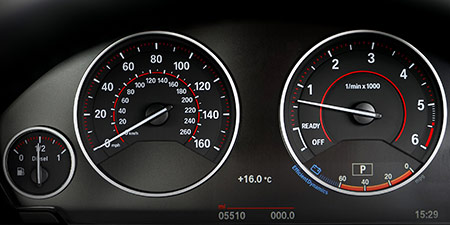# Kilometers per hour (km/h) to Miles per hour (mph)

Units of speed ﹣ Converter Kilometers per hour to Miles per hour

Here you can convert the Speed unit Kilometers per hour into the unit Miles per hour and vice versa you can convert Miles per hour into Kilometers per hour. By clicking the "Swap units" icon, you will always obtain the desired conversion in the calculation result, i.e., km/h to mph or mph to km/h. With the following calculator you can also calculate any other Speed unit.

## Info about "Kilometers per hour"

Kilometers per hour is used as a standard unit in transportation, therefore it is used in traffic or to measure the travel speed of vehicles. For example, a commercial aircraft flies at a speed of 1,000 km/h then it covers a distance of 1,000 kilometers per hour.

The unit is defined as follows: An object moving at 1 "km/h" per hour will cover one kilometer. "km/h" is approved to use in the international system of units (SI).

## Info about "Miles per hour"

The unit of length known as a "mile" and it should not be confused with the nautical mile and very commonly used in the Anglo-American countries. Therefore, the metric system is defined in miles (instead of kilometers) per time unit. For example, if a vehicle travels at a speed of 100 miles per hour (100 mph), it will move around 160 km per hour in the metric system.

The units are defined as follows: An object moving at 1 "mph" per hour will cover a distance of one mile. Therefore, 1 mile is equivalent to 1.609344 kilometers.

## Basis for conversion Kilometers per hour (km/h) to Miles per hour (mph) and vice versa

The abbreviation for the "Speed unit Kilometers per hour" is km/h. The abbreviation for the "Speed unit Miles per hour" is mph.

## Formula for the conversion of Kilometers per hour (km/h) to Miles per hour (mph) and vice versa

The calculation from Kilometers per hour to Miles per hour shall be made using the following conversion formula:

Conversion formula Kilometers per hour to Miles per hour

Determine the number of Miles per hour from Kilometers per hour

Kilometers per hour × 0.62137119248733

## Formula for the conversion of Miles per hour (mph) to Kilometers per hour (km/h)

The calculation from Miles per hour to Kilometers per hour shall be made using the following conversion formula:

Conversion formula Miles per hour to Kilometers per hour

Determine the number of Kilometers per hour from Miles per hour

Miles per hour × 1.6093439993525

## Overview table : How many Kilometers per hour are in a Miles per hour ?

Kilometers per hour km/h ⇒ Miles per hour mph
0.01 km/h  are  0.00621 mph
0.02 km/h  are  0.01242 mph
0.03 km/h  are  0.01864 mph
0.04 km/h  are  0.02485 mph
0.05 km/h  are  0.03106 mph
0.06 km/h  are  0.03728 mph
0.07 km/h  are  0.04349 mph
0.08 km/h  are  0.04971 mph
0.09 km/h  are  0.05592 mph
0.10 km/h  are  0.06213 mph
0.20 km/h  are  0.12427 mph
0.30 km/h  are  0.18641 mph
0.40 km/h  are  0.24854 mph
0.50 km/h  are  0.31068 mph
0.60 km/h  are  0.37282 mph
0.70 km/h  are  0.43496 mph
0.80 km/h  are  0.49709 mph
0.90 km/h  are  0.55923 mph
1 km/h  corresponds to  0.62137 mph
2 km/h  are  1.24274 mph
3 km/h  are  1.86411 mph
4 km/h  are  2.48548 mph
5 km/h  are  3.10685 mph
6 km/h  are  3.72822 mph
7 km/h  are  4.34959 mph
8 km/h  are  4.97097 mph
9 km/h  are  5.59234 mph
10 km/h  are  6.21371 mph
20 km/h  are  12.42742 mph
30 km/h  are  18.64113 mph
40 km/h  are  24.85484 mph
50 km/h  are  31.06856 mph
60 km/h  are  37.28227 mph
70 km/h  are  43.49598 mph
80 km/h  are  49.70969 mph
90 km/h  are  55.92340 mph
100 km/h  are  62.13711 mph
200 km/h  are  124.27423 mph
300 km/h  are  186.41135 mph
400 km/h  are  248.54847 mph
500 km/h  are  310.68559 mph
600 km/h  are  372.82271 mph
700 km/h  are  434.95983 mph
800 km/h  are  497.09695 mph
900 km/h  are  559.23407 mph
1 000 km/h  are  621.37119 mph

## Overview table : How many Miles per hour are in a Kilometers per hour ?

Miles per hour mph ⇒ Kilometers per hour km/h
0.01 mph  are  0.01609 km/h
0.02 mph  are  0.03218 km/h
0.03 mph  are  0.04828 km/h
0.04 mph  are  0.06437 km/h
0.05 mph  are  0.08046 km/h
0.06 mph  are  0.09656 km/h
0.07 mph  are  0.11265 km/h
0.08 mph  are  0.12874 km/h
0.09 mph  are  0.14484 km/h
0.10 mph  are  0.16093 km/h
0.20 mph  are  0.32186 km/h
0.30 mph  are  0.48280 km/h
0.40 mph  are  0.64373 km/h
0.50 mph  are  0.80467 km/h
0.60 mph  are  0.96560 km/h
0.70 mph  are  1.12654 km/h
0.80 mph  are  1.28747 km/h
0.90 mph  are  1.44841 km/h
1 mph  corresponds to  1.60934 km/h
2 mph  are  3.21868 km/h
3 mph  are  4.82803 km/h
4 mph  are  6.43737 km/h
5 mph  are  8.04672 km/h
6 mph  are  9.65606 km/h
7 mph  are  11.26540 km/h
8 mph  are  12.87475 km/h
9 mph  are  14.48409 km/h
10 mph  are  16.09344 km/h
20 mph  are  32.18688 km/h
30 mph  are  48.28032 km/h
40 mph  are  64.37376 km/h
50 mph  are  80.46720 km/h
60 mph  are  96.56064 km/h
70 mph  are  112.65408 km/h
80 mph  are  128.74752 km/h
90 mph  are  144.84096 km/h
100 mph  are  160.93440 km/h
200 mph  are  321.86880 km/h
300 mph  are  482.80320 km/h
400 mph  are  643.73760 km/h
500 mph  are  804.67200 km/h
600 mph  are  965.60640 km/h
700 mph  are  1 126.54080 km/h
800 mph  are  1 287.47519 km/h
900 mph  are  1 448.40959 km/h
1 000 mph  are  1 609.34399 km/h

## Source information

As source for the information in the 'Units of speed' category, we have used in particular:

## Last update on February 19, 2023

The pages of the 'Units of speed' category were last editorially reviewed by Stefan Banse on February 19, 2023. They all correspond to the current status.

### Previous changes on October 9, 2021

• November 9, 2020: Publication of the Speed converter
• Editorial revision of all texts in this category